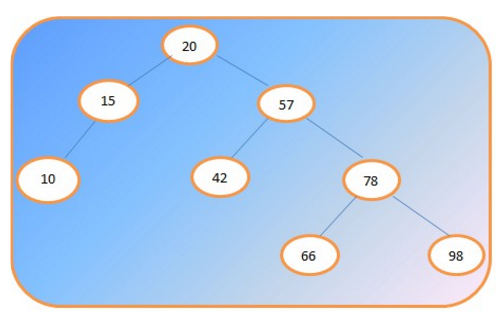# In - Order Traversal sequence of Binary Tree - Data Structure

Q.  Which of the following techniques represents the precise sequence of an In - Order Traversal of a Binary Tree?
- Published on 27 Aug 15

a. Visit the Root, Traverse Left Subtree, Traverse Right Subtree
b. Traverse Left Subtree, Visit the Root, Traverse Right Subtree
c. Traverse Left Subtree, Traverse Right Subtree, Visit the Root
d. None of the Above

ANSWER: Traverse Left Subtree, Visit the Root, Traverse Right Subtree

#### Discussion

• Nirja Shah   -Posted on 18 Nov 15
Traversing a binary tree means visiting each node of a tree exactly once. Traversal of tree gives the linear order of nodes. There are three types of traversal in binary tree. Preorder, Inorder and Postorder traversal.
Algorithm for in order traversal.
- Traverse left subtree of root in inorder.
- Visit the Root.
- Traverse right subtree of root in inorder.
Consider the following example.In-order traversal of above binary tree is:
10 15 20 42 57 66 78 98

## ➨ Post your comment / Share knowledgeEnter the code shown above:

(Note: If you cannot read the numbers in the above image, reload the page to generate a new one.)